# The Art of Electronics - SINGLE ENDED FOLLOWER AMPLIFIER (pg.91)

#### vol_

Joined Dec 2, 2015
93
This is a schematic from the book The Art of Electronics, pg. 91: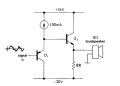The text describing the amplifier is the following: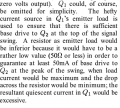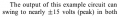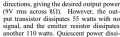My questions are:

1) How a 50 ohm emitter resistor on Q1 can guarantee for 50mA Q2 base current?
2) How can it be the output transistor to dissipate 55 W (i'm getting 45 W in my calculations) and the emitter resistor to dissipate 110 W???
3) Why the output of this example circuit swing to nearly +/- 15 V???

#### Jony130

Joined Feb 17, 2009
5,439
1) How a 50 ohm emitter resistor on Q1 can guarantee for 50mA Q2 base current?
At the peak Q1 is Cut-off so for Pmax = 10W we have VL_max = √(10W *8Ω) ≈ 9V rms---->12.7Vpeak, therefore the voltage at base is larger than 13V.
Rb = (15V - 13V)/50mA = 2V/50mA = 40Ω.
How can it be the output transistor to dissipate 55 W (i'm getting 45 W in my calculations) and the emitter resistor to dissipate 110 W???
Show as your calculation. As for power across Re the case is simple P = U^2 *R = 15V^2*8Ω = 112.5W ≈ 110W.
Why the output of this example circuit swing to nearly +/- 15 V???
What voltage at the Q2 base will cut-off at Q2 transistor?

Last edited:
•vol_

#### vol_

Joined Dec 2, 2015
93
1) Jony thanks a lot! I would like to buy you a six pack of beers, but i cant afford the shipping expenses.

2) Excuse me but for the emitter resistor power is: P = U^2 /R and not U^2*R. So, because we have a swing of +/- 15V the power for Re will be: P=30V^2/8Ω = 112,5W, thats almost 110W. Rounding the number down because we are expecting loses in the rails and the Q2 transistor (?)...
And for the Q2 transistor the quiescent current at the peak of the output is: Iq=sqrt(P/R)=sqrt(10/8)=1,2A (although 1,2A its kind of cooked to match the text numbers. The answer i would use is 1,1A. Or we round up because we expect loses again?). And also i cant really understand why we don't take into account the voltage drop occurring at the emitter and load resistors parallel combination???
So the power dissipation will be: P=V*I=45V * 1,2A=54W, and thats almost 55W.

3) What voltage at the Q2 base will cut-off at Q2 transistor?[/QUOTE]
Given that the signal is ground referenced we can see that:
The emitter resistor and the load resistor form a voltage divider, so the output peak negative swing will be -30V /2 = -15V
The output peak positive swing will be +15V, +14,8V actually or else Q2 will be saturated. (Question here: so compliance its the saturation voltage of the collector?)

#### vol_

Joined Dec 2, 2015
93
Note that i used some bold question marks for some new minor question i have. Thanks every one!

#### vol_

Joined Dec 2, 2015
93
And also the output voltage swing is independent the desired output power as we analyzed the circuit so far!?!?
The only thing that the output goals influence is the Q2 base current..
Is that so?

#### Jony130

Joined Feb 17, 2009
5,439
2) Excuse me but for the emitter resistor power is: P = U^2 /R and not U^2*R. So, because we have a swing of +/- 15V the power for Re will be: P=30V^2/8Ω = 112,5W, thats almost 110W.
We do not need to know the voltage swing across Re to be able to find emitter resistor power. All we need to know is a DC condition (Vin_AC = 0V) and quiescent current. Therefore IcqQ1 = 100mA and IcqQ2 = Vee/Re = 30V/8Ω = 3.75A. From there we can find power dissipated in Re.
P = V*I = 30V*3.75A = 112.5W. We do the same thing for Q2 (we can do that because Q2 operates in Class A) Pd = Vce*Icq = 15V*3.75A = 56.25W.

The emitter resistor and the load resistor form a voltage divider, so the output peak negative swing will be -30V /2 = -15V
The output peak positive swing will be +15V, +14,8V actually or else Q2 will be saturated.
Very good. But what about this current source at Q2 base? Can this current source be able to provide 100mA of a base current if the voltage across it is 0.2V or less? Also notice that if Q2 current gain is 50 the Ie_max = 100mA * 51 = 5.1A, so the output peak positive swing will be (5.1A*4Ω)+ (-30V)*4Ω/8Ω = 20.4V + (-15V) = 5.4Vpeak . And the output power P = VL_peak^2/(2RL) = 5.4V^2/16Ω = 1.8W

#### vol_

Joined Dec 2, 2015
93
Also notice that if Q2 current gain is 50 the Ie_max = 100mA * 51 = 5.1A, so the output peak positive swing will be (5.1A*4Ω)+ (-30V)*4Ω/8Ω = 20.4V + (-15V) = 5.4Vpeak . And the output power P = VL_peak^2/(2RL) = 5.4V^2/16Ω = 1.8W
So we need a current gain of more than 70 to get 10Wrms output? Is that what you want to clarify?

As for the voltage at the Q2 base that will cut-off the Q2 transistor I really cant figure it out..

Thanks for your clear and intuitive way.

#### Jony130

Joined Feb 17, 2009
5,439
As for the voltage at the Q2 base that will cut-off the Q2 transistor I really cant figure it out..
But you're almost there. "The emitter resistor and the load resistor form a voltage divider, so the output peak negative swing will be -30V /2 = -15V"
This means that any voltage more negative than -14.4V will start cut-off Q2.

#### vol_

Joined Dec 2, 2015
93
I still dont get it about Q2's cut off stage..
So having that the emitter follower has current gain I should expect the signal source to have a swing of +/- 15V to have an output swing of +/- 15V. Is that correct?
So for a source voltage larger than -14,4V we will got clipping and the Q2 will cut off. So for a voltage of 0,6V and smaller across the current source, the current source wont give any current to the circuit. I hope I'm right this time..And what about the current gain? Do I need it to be larger than 70 to have a 10Wrms output?

Also is compliance referring to saturation?

I hope I am not asking too much.. But I want to demystify this world in my head..

Thanks for everything. You and the other guys with your replies help me to put the foundations in understanding basic electronics. Without these replies i would be floating on a small dc current forever.. I also really enjoy Horowitz and Winfield way of thinking and describing, although I am struggling really hard to read a single page of their book...

Cheers everyone!

#### Jony130

Joined Feb 17, 2009
5,439
Try exam this circuit (Figure 2.8 and 2.9 in AoE)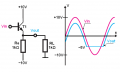Can you explain why the output voltage is "clip" at -5V ?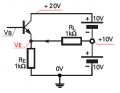Can you find the voltage needed at base to Turn-ON this transistor? Do you see similarities?

Last edited:
•vol_

#### vol_

Joined Dec 2, 2015
93
Can you explain why the output voltage is "clip" at -5V ?
If Vb<-4,4V, -6V for example, we would get: Vb=-6V > Ve=-5V, with collector voltage Vc=10V. So the b-e junction bias would be reverse biased with the c-e junction reverse biased. So we are in the cut-off stage (Ve>Vb<Vc).

Can you find the voltage needed at base to Turn-ON this transistor? Do you see similarities?
So, doing as before, I conclude that Vb should be larger than 5,6V to have the transistor in the active region of operation. And the output swing will be +5V to +19,8V.

I really thank everyone for the time you're devoting in this forum.. I am grateful!

#### Jony130

Joined Feb 17, 2009
5,439
Good job, but why the output will swing w up to +19.8V ? If Vout is 19.8V Vin must be equal to 19.8V + 0.7V = 20.5V greater than Vcc.

•vol_

#### vol_

Joined Dec 2, 2015
93
Good job, but why the output will swing w up to +19.8V ? If Vout is 19.8V Vin must be equal to 19.8V + 0.7V = 20.5V greater than Vcc.
Good question again. The transistor will be in the active state until Vout reaches 19,4V. For greater output voltages, 19,5V for example we will have voltages of: Ve=19,5V < Vb=20,1V > Vc=20V. The transistor will be saturated (Ve<Vb>Vc), Vb>20V cant happen, and the transistor asking for greater output voltage will sink a little bit of current from the emitter resistor putting the maximum output voltage to within a saturation voltage drop of Vcc (19,8V - 19,9V).

This is what i understand from AoE pg. 67, where also figure 2.8 is shown.

Cheers!

#### Jony130

Joined Feb 17, 2009
5,439
If Vb can reach Vcc max (20V). The BJT will still be in active region. Vb = 20V, Vc = 20V and Ve = 19.4V therefore Vbc = 0V so, no saturation.
BJT will enter satiation region only if Vbc > 0.3...0.5V.

Last edited:

#### vol_

Joined Dec 2, 2015
93
Vb = 20V, Vc= 10 and Ve = 19.4V therefore Vbc = 0V
You mean Vc=20V? I hope you do.. Because if Vb=20V and Vc=10V, Vbc=10V. If you have it correct then Ve<Vb>Vc, so saturation will occur.

#### Jony130

Joined Feb 17, 2009
5,439

#### Jony130

Joined Feb 17, 2009
5,439
For Vout = Ve = 9.9V we have Vc = 10V and Vb = 0.6+Ve = 10.5V witch means that Vbc = 10.5V - 10V = 0.5V and this result means that base-collector junction is forward biased, and this is why BJT is saturated.

#### vol_

Joined Dec 2, 2015
93
Good job, but why the output will swing w up to +19.8V ? If Vout is 19.8V Vin must be equal to 19.8V + 0.7V = 20.5V greater than Vcc.
So also here we will have saturation? Is there something wrong in this situation?

Thanks mate..

#### Jony130

Joined Feb 17, 2009
5,439
So also here we will have saturation?
Yes, we are one edge of saturation.
Is there something wrong in this situation?
There is nothing wrong in saturation, but in saturation BJT loses all his current gain which is not good for our amplifier. But you probably know this already.

•vol_